Photon energies in a spherical body with va=.6 ca      The photon pattern is symmetrical and we will focus on the 0 to 180 degree half. When photons are emitted, they are blueshifted or redshifted by a factor of ca/(ca−velocity of source in direction of photon motion through qm) or ca/(ca−va·cosα) where va is the velocity of the system through the qm and α is the angle between the photon's path through the qm and va or the x axis. For photons moving in the +x direction through the system, cosα=1 so the blueshift is simply 1/(1−.6) or 2.5. Therefore, the photons moving from the sphere's center to the 0° location have a frequency equal to the emission frequency times the blueshift or (.8·2.5) or 2 times the at-rest frequency. Therefore, their energy is also 2 times the at-rest energy or 2 ep, as shown in the table. For photons moving in the −x direction, cosα=−1 and at emission the photons are redshifted by a factor of 1/(1+.6) or .625, and they have an energy of (.8·.625) or .5 times the at-rest energy or .5 ep, as shown.Photons moving from the source to the 90° location on the sphere travel through the qm at an angle of 53.1° to the x axis (just as photons in the light clocks oriented in the y direction were traveling through the qm at 53.1° to the x axis). Therefore, they are blueshifted by a factor of 1.5625. They have a frequency of .8·1.5625 or 1.25 times the at-rest frequency and an energy of 1.2500 ep. Similarly, photons moving from the center to the θ=30° location (absolute angle θa=35.8°) move at 15.2° through the qm and they have an energy of 1.8995 ep.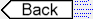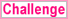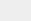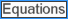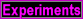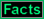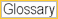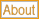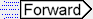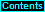To page: 1 2 3 3a 4 5 6 7 8 9 10 11 12 13 14 15 16 17 18 19 20 21 22 23 24 25 26 27 28 29 29a 30 31 32